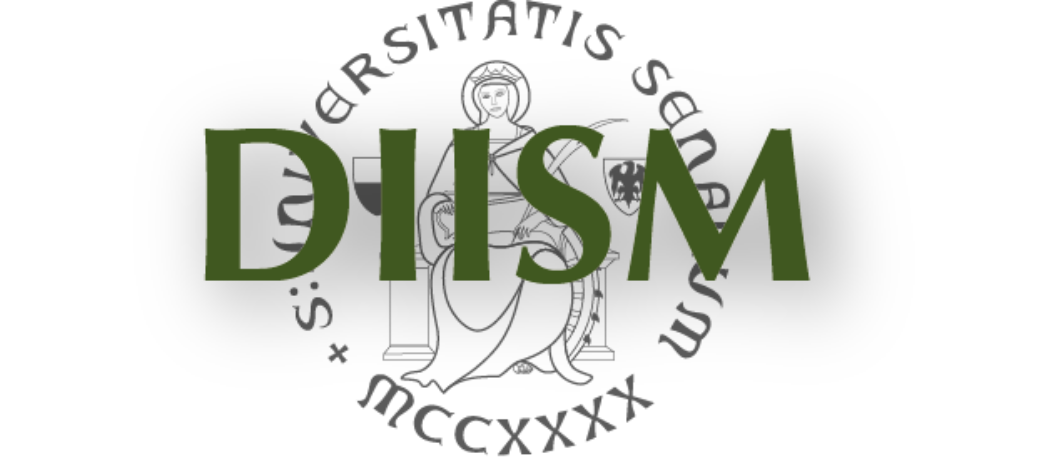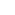DIPARTIMENTO DI INGEGNERIA DELL'INFORMAZIONEE SCIENZE MATEMATICHE UNIVERSITA' DEGLI STUDI DI SIENAPrivacy e Cookie policy

 EducationCorsi di studioInsegnamenti

InsegnamentoMathematical methods for engineering a.a. 2013-2014 Corso Lm Computer and Automation EngineeringLm Matematica Anno 1 Semestre II Obiettivi To master advanced mathematical tools in engineering, through the study of basic topics in functional analysis, differential equations and complex functions, with emphasis on current applications. Ore 81 Crediti 9 Docente Stefano Campi
 Programma Normed spaces: metric and topological properties. Banach spaces. The contraction mapping theorem. Spaces of continuous and differentiable functions. Application: global existence and uniqueness theorem for Cauchy problems. Inner product spaces. Hilbert spaces. Orthogonal projections. Generalized Fourier series. A complete integral: introduction to the generalized Riemann integral. Monotone and dominated convergence theorems. Hints on Lp-spaces. Trigonometric Fourier series and their convergence. Linear differential equations. Structure of the solution set. Sturm-Liouville problems. The homogeneous case: self-adjointness, eigenvalues, eigenfunctions. The non-homogeneous case: Fourier series expansion of the solutions. Green's functions. Hints on partial differential equations: separation of variables techniques. Complex numbers: algebraic and polar forms. Complex differentiation and its consequences. Olomorphic functions. Harmonic functions. Elementary complex functions: the exponential and the logarithms, trigonometric and hyperbolic functions, root functions. Power series and analytic functions. Complex integration. Primitives. Cauchy's Theorem and its consequences. Laurent series. Isolated singularities. Residues. Complex integration techniques for the evaluation of real integrals. Hints on branching singularities.

 Dipartimento di Ingegneria dell'Informazione e Scienze Matematiche - Via Roma, 56 53100 SIENA - Italy